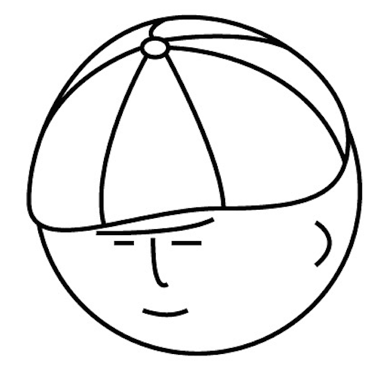マツシタのお勉強メモ

マツシタのお勉強

welcome to my engineering blog
Hacker RankWhat is Bucket Sort

Bucket Sort is linear sort algorithm. It takes O(N) time. But this algorithm has some restrictions to use.

The restrictions are following

• We know the maximum value in array
• The maximum value is not too large
• each element is Integer .

In Bucket sort, we have to array called bucket that the size is max value in array. So if the max value is too large, we cannot create the array because we would get OutOfMemoryError. And each element in array will be index of array, so each element have to be Integer.

Structure of Bucket Sort

Bucket sort is implemented by using array like this.

1. Prepare a array that the size of array is max value in inputted array
2. Put element into the position of array called bucket where element is index.
3. Take elements from bucket in order.

Complexity

Time complexity is 0(N). This is very fast. Space complexity is O(max value). This is not good point.